# 数据科学在量化金融中的应用：指数预测（下）

01/12 16:53## 特征工程

• 处理缺失值并提取所需变量
• 数据标准化
• 处理分类变量

### 1.  处理缺失值并提取所需变量

x_input = (df_model.dropna()[['Year','Month','Day','Weekday','seasonality','sign_t_1','t_1_PricePctDelta','t_2_PricePctDelta','t_1_VolumeDelta']].reset_index(drop=True))
x_input.head(10)y = df_model.dropna().reset_index(drop=True)['AdjPricePctDelta']

### 2.  数据标准化

scaler = StandardScaler()
x = x_input.copy()
x[['t_1_PricePctDelta','t_2_PricePctDelta','t_1_VolumeDelta']]=scaler.fit_transform(x[['t_1_PricePctDelta','t_2_PricePctDelta','t_1_VolumeDelta']])

### 3.  处理分类变量

x_mod = pd.get_dummies(data=x, columns=['Year','Month','Day','Weekday','seasonality'])
x_mod.columns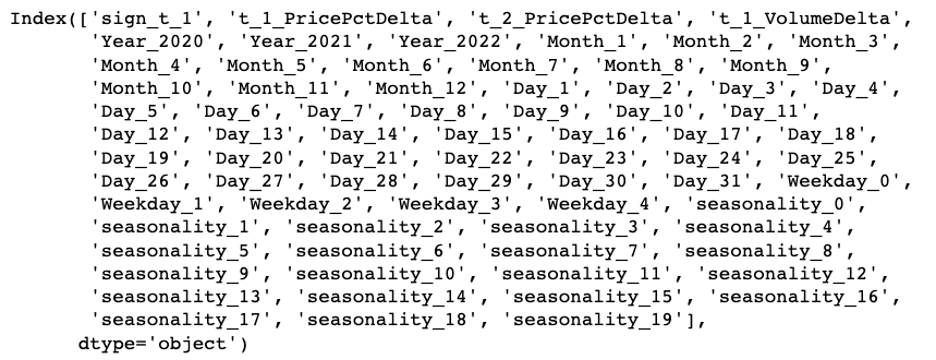x_mod.shape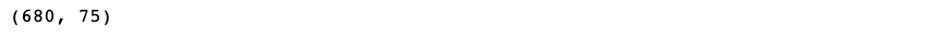## 模型评估

trail_result = ensemble_method_reg_trails(x_train, y_train, x_test, y_test)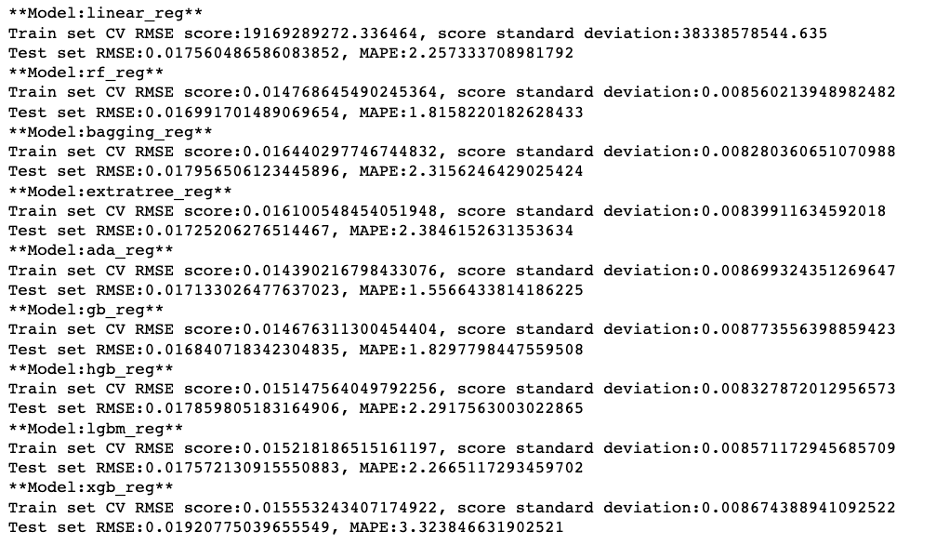pd.DataFrame(trail_result).sort_values('model_test_mape', ascending=True)• 使用 RandomizedSearchCV 寻找最佳参数的大致范围
• 使用 GridSearchCV 寻找更精确的参数

• n_estimators
• base_estimator
• learning_rate

base_estimator 是 ada boost 提升算法的基础，我们需要提前建立一个 base_estimator 的列表。

l_base_estimator = []
for i in range(1,16):
base = DecisionTreeRegressor(max_depth=i, random_state=42)
l_base_estimator.append(base)
l_base_estimator += [LinearSVR(random_state=42,epsilon=0.01,C=100)]

### 1.  使用 RandomizedSearchCV 寻找最佳参数的大致范围

randomized_search_grid = {'n_estimators':[10, 50, 100, 500, 1000, 5000],
'base_estimator':l_base_estimator,
'learning_rate':np.linspace(0.01,1)}
search = RandomizedSearchCV(AdaBoostRegressor(random_state=42),
randomized_search_grid,
n_iter=500,
scoring='neg_mean_absolute_error',
n_jobs=-1,
cv=5,
random_state=42)
result = search.fit(x_train, y_train)

result.best_params_result.best_score_### 2.  使用 GridSearchCV 寻找更精确的参数

• n_estimators: 1-50
• base_estimator: Decision Tree with max depth 9
• learning_rate: 0.7左右
search_grid = {'n_estimators':range(1,51),
'learning_rate':np.linspace(0.6,0.8,num=20)}GridSearchCV 的结果如下：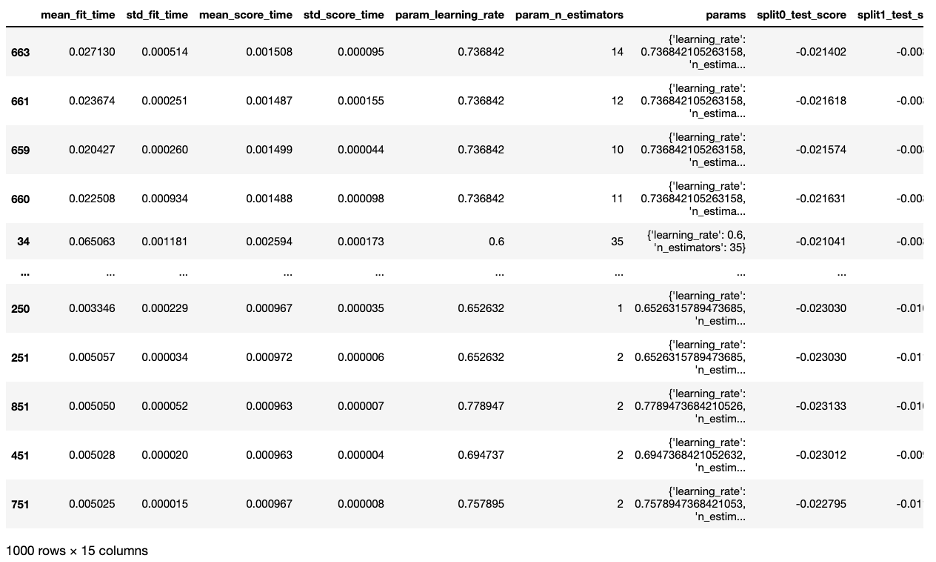best_reg.fit(x_mod, y)m_forecast = best_reg.predict(x_mod)
mean_absolute_percentage_error(y, m_forecast)## 模型预测

def forecast_one_period(price_info_adj_data, ml_model, data_processor):
# Source data: Data acquired straight from source
next_day = last_record['Date'] + relativedelta(days=1)
next_day_t_2_PricePctDelta = last_record['t_1_PricePctDelta']
next_day_t_1_VolumeDelta = last_record['Volume_in_M'] - last_record['t_1_VolumeDelta']
if next_day_t_1_PricePctDelta > 0:
next_day_sign_t_1 = 1
else:
next_day_sign_t_1 = 0
# Value -99999 is a placeholder which won't be used in the following modeling process
next_day_input = (pd.DataFrame({'Date':[next_day],
'Volume_in_M':[-99999],
't_1_PricePctDelta':[next_day_t_1_PricePctDelta],
't_2_PricePctDelta':[next_day_t_2_PricePctDelta],
't-1volume': last_record['Volume_in_M'],
't-2volume': last_record['t-1volume'],
't_1_VolumeDelta':[next_day_t_1_VolumeDelta],
'sign_t_1':next_day_sign_t_1}).set_index('Date'))
# If forecast period is post Feb 15, 2020, input data starts from 2020-02-16,
# as our model is dedicated for market under Covid Impact.
# Another model could be used for pre-Covid market forecast.
if next_day > datetime.datetime(2020, 2, 15):
# Add new record to original data for modeling preparation
# Prep for modeling
x,y = data_processor.data_modeling_prep(input_modified)
next_day_x = x.iloc[-1:]
forecast_price_delta = ml_model.predict(next_day_x)
# Consolidate prediction results
forecast_df = {'Date':[next_day], 'price_pct_delta':[forecast_price_delta], 'actual_pct_delta':[np.nan]}
return pd.DataFrame(forecast_df)## 分析预测结果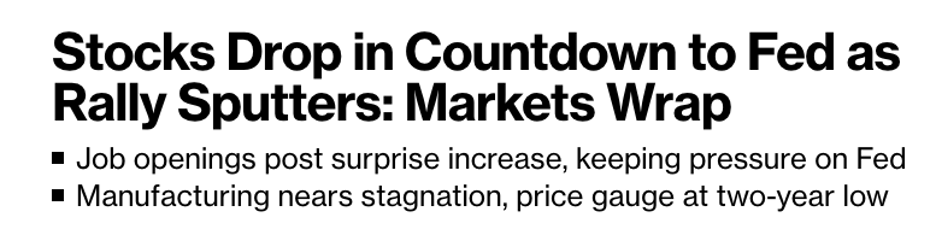“Stocks finished lower as data showing a solid US labor market bolstered speculation that Federal Reserve policy could remain aggressively tight even with the threat of a recession.”

## 总结

• 确定预测目标：反映北美股票市场的指数 — 标普500 ；
• 数据收集：从公共金融网站下载历史价格数据；
• 探索性数据分析：初步了解数据的特性，数据可视化，将时间序列信息以图像的形式呈现；
• 数据预处理：将时间转换为变量，更改价格数据，寻找周期和季节性，根据周期调整交易量数据；
• 数据工程：处理缺失值并提取所需变量，数据标准化，处理分类变量；
• 模型选择和训练：拆分训练集和测试集，确定模型方向和评估指标，尝试训练各种模型；
• 模型评估：根据指标选定最优模型，使用 RandomizedSearchCV 寻找最佳参数的大致范围，再使用 GridSearchCV 寻找更精确的参数；
• 模型预测：整合输入数据，预测未来一个工作日的价格变动；
• 分析预测结果：结合当日的实际情况，理解市场变动，发挥模型价值。### 参考资料：### 作者的其它热门文章

0
3 收藏

0 评论
3 收藏
0